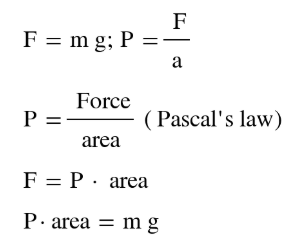# Problem: The gravitational force exerted by an object is given by F = mg, where F is the force in newtons, m is the mass in kilograms, and g is the acceleration due to gravity (9.81 m/s 2). (a) Use the definition of the pascal to calculate the mass (in kg) of the atmosphere above 1 m2 of ocean. (1 Pa = 1.01325x105 N/m2)

###### FREE Expert Solution
• From the equation F = mg, we need to find the actual force exerted by the atmosphere.
• Force can also be calculated using the formula Pressure = F / area
• Combine both equations to get the mass using the pressure and the given area
• 1 atm can be equated to 1.01325x105 Pascals• Therefore the atmosphere at this level can be assumed having a force of 1.01325x105 Pa * 1 m2
• Now plug in this to the P•area = mg equation
81% (293 ratings)###### Problem Details

The gravitational force exerted by an object is given by F = mg, where F is the force in newtons, m is the mass in kilograms, and g is the acceleration due to gravity (9.81 m/s 2).

(a) Use the definition of the pascal to calculate the mass (in kg) of the atmosphere above 1 m2 of ocean. (1 Pa = 1.01325x105 N/m2)